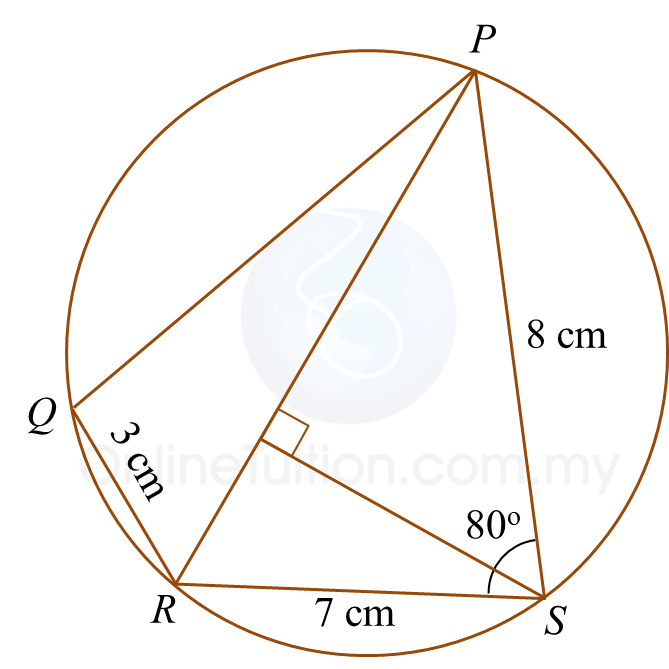# 10.4.4 Solution of Triangles, Long Questions (Question 7 & 8)

Question 7:
Diagram below shows a quadrilateral ABCD where the sides AB and CD are parallel. ∠BAC is an obtuse angle.Given that AB = 14 cm, BC = 27 cm, ∠ACB = 30o and AB : DC = 7 : 3.
Calculate
(a) ∠BAC.
(b) the length, in cm, of diagonal BD.
(c) the area, in cm2, of quadrilateral ABCD.

Solution:
(a)

(b)

(c)

Question 8:
Diagram below shows a cyclic quadrilateral PQRS.(a) Calculate
(i) the length, in cm, of PR,
(ii) ∠PRQ.
(b) Find
(i) the area, in cm2, of ∆ PRS,
(ii) the short distance, in cm, from point S to PR.

Solution:
(a)(i)

(a)(ii)

(b)(i)

(b)(ii)